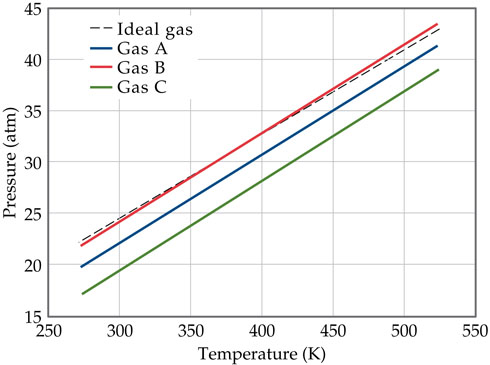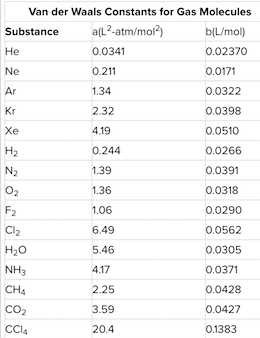# Problem: The graph below shows the change in pressure as the temperature increases for a 1-mol sample of a gas confined to a 1-L container. The four plots correspond to an ideal gas and three real gases: CO2, N2, and Cl2.Use the van der Waals constants in the table below to match the labels in the plot (A, B, and C) with the respective gases (CO2, N2, and Cl2).

###### FREE Expert Solution
95% (204 ratings)
###### Problem Details

The graph below shows the change in pressure as the temperature increases for a 1-mol sample of a gas confined to a 1-L container. The four plots correspond to an ideal gas and three real gases: CO2, N2, and Cl2.Use the van der Waals constants in the table below to match the labels in the plot (A, B, and C) with the respective gases (CO2, N2, and Cl2).What scientific concept do you need to know in order to solve this problem?

Our tutors have indicated that to solve this problem you will need to apply the Van der Waals Equation concept. You can view video lessons to learn Van der Waals Equation. Or if you need more Van der Waals Equation practice, you can also practice Van der Waals Equation practice problems.

What is the difficulty of this problem?

Our tutors rated the difficulty ofThe graph below shows the change in pressure as the temperat...as medium difficulty.

How long does this problem take to solve?

Our expert Chemistry tutor, Dasha took 7 minutes and 36 seconds to solve this problem. You can follow their steps in the video explanation above.

What professor is this problem relevant for?

Based on our data, we think this problem is relevant for Professor Bliem's class at UNC.